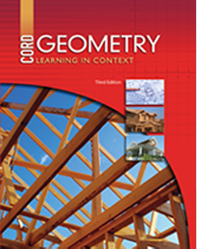# Geometry - Third Edition

## Chapter 7: Coordinate Geometry

Some links are repeated for use with more than one lesson.

Please report broken links to Kathy Kral

### This site applies to the entire chapter.

http://www.math.com/school/subject3/lessons/S3U1L2GL.html
This site discusses coordinate geometry.

### 7.1 Distance on the Coordinate Plane

http://www.purplemath.com/modules/distform.htm
This page explains the distance formula, shows explains, and discusses common mistakes students make when using it.

http://www.explorelearning.com/index.cfm?method=cResource.dspDetail&ResourceID=64
This page contains a gizmo exploring the distance formula.

### 7.2 Vectors on the Coordinate Plane

http://www.phy6.org/stargaze/Svector.htm
This page explains vectors and many different components of them.

http://hyperphysics.phy-astr.gsu.edu/hbase/vect.html
This page explains vectors and basic vector operations.

### 7.3 The Slope Formula

http://cs.selu.edu/~rbyrd/math/slope/
This page states the slope formula and contains an example problem with a solution.

http://www.regentsprep.org/regents/math/algebra/ac1/indexAC1.htm
NEW This page has links to lessons and practice problems about the slope of a line, spanning topics for this and the next lesson.

### 7.4 Linear Equations

http://www.regentsprep.org/regents/math/algebra/ac1/indexAC1.htm
NEW This page has links to lessons and practice problems about the slope of a line, spanning topics for this and the previous lesson.

http://edhelper.com/LinearEquations16.htm
This page is a sample worksheet of problem for writing an equation of the line.

### 7.5 Coordinate Proof

http://www.regentsprep.org/regents/math/geometry/GCG4/indexGCG4.htm
NEW This page discusses and provides practice with proofs in coordinate geometry.

### 7.6 Coordinates in Space

http://www.uni-duesseldorf.de/URZ/hardware/parallel/local/softimage/Soft3D_html/3d_learn/bas/b_coor.htm
This page explains the Cartesian coordinate system using X, Y, and Z and how to find points in space.